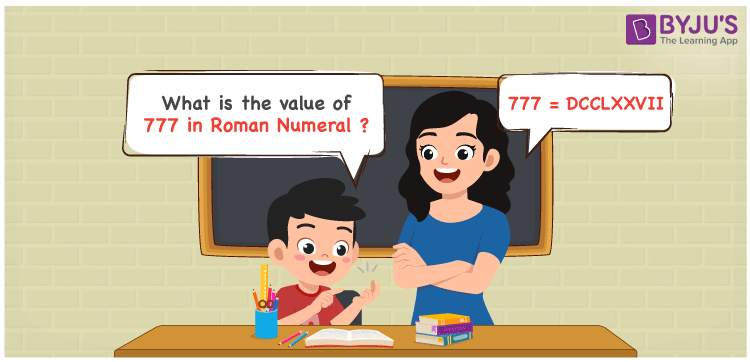# 777 in Roman Numerals

777 in Roman Numerals is DCCLXXVII. 777 is an arabic number that can be represented by Roman letters. In this article, learn how 777 can be shown in Roman numerals in a systematic manner. The faculty use simple language to explain the steps so that students can learn it without further confusion. Therefore, the number 777 is written in roman numerals as DCCLXXVII.

 Number Roman Numeral 777 DCCLXXVII## How to Write 777 in Roman Numerals?

Learn the expansion and conversion of 777 to DCCLXXVII using the steps given here.

777 = 500 + 100 + 100 + 50 + 10 + 10 + 5 + 1 + 1

777 = D + C + C + L + X + X + V + I + I

777 = DCCLXXVII

## Video Lesson on Roman Numerals## Frequently Asked Questions on 777 in Roman Numerals

Q1

### Write 777 in Roman Numerals.

The transformation of 777 in Roman numerals uses the place value chart which shows the ones, tens and hundreds value.
Hundreds = 700 = DCC
Tens = 70 = LXX
Ones = 7 = VII
Number = 700 + 70 + 7 = DCCLXXVII
Q2

### Find 700 + 77.

We know that
700 + 77 = 777
Therefore, 700 + 77 is 777 which can be written as DCCLXXVII.
Q3

### Show the conversion of 777 to Roman Numerals.

In roman numerals,
777 = 500 + 100 + 100 + 50 + 10 + 10 + 5 + 1 + 1
777 = D + C + C + L + X + X + V + I + I
777 = DCCLXXVII